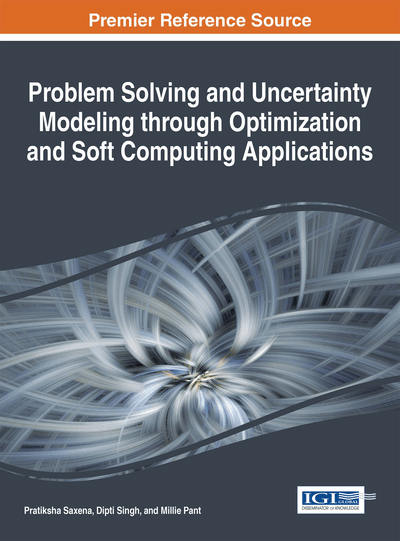# Determination of Bearing Capacity of Shallow Foundation Using Soft Computing

Jagan J. (VIT University, India), Swaptik Chowdhury (VIT University, India), Pratik Goyal (VIT University, India), Pijush Samui (NIT Patna, India) and Yıldırım Dalkiliç (Erzincan University, Turkey)
DOI: 10.4018/978-1-4666-9885-7.ch014

## Abstract

The ultimate bearing capacity is an important criterion for the successful implementation of any geotechnical projects. This chapter studies the feasibility of employing Gaussian process regression (GPR), Extreme learning machine (ELM) and Minimax probability machine regression (MPMR) for prediction of ultimate bearing capacity of shallow foundation based on cohesionless soils. The developed models have been compared on the basis of coefficient of relation (R) values (GPR= 0.9625, ELM= 0.938, MPMR= 0.9625). The results show that MPMR is more efficient tool but the models of GPR and ELM also gives satisfactory results.
Chapter Preview
Top

## Introduction

Every foundation design should necessarily satisfy two major criteria of ultimate bearing capacity and limiting settlement of foundation for its successful application in construction (Beer, 1965). The ultimate bearing capacity depends upon the shearing strength of the soil and is primarily determined from the theories proposed by Terzaghi (1943), Meyerhof (1963), Vesic (1973), among others. The basic principle of the bearing capacity theories is the limit equilibrium and the values which of the bearing capacities which are obtained, are validated through the experimental researches. However, the major disadvantage of these researches is that they are generally done on highly scaled down models of real footings because the shearing strain along the slip line increases with the increase in width of foundation and the ratio of mean grain size and the footing width (Steenfelt, 1977). .Although the scale effect becomes insignificant when the ratio of the mean grain size and the width of footing are less than a certain limit which is decided accordingly to the soil types (Tatsuoka et al., 1991). Hence the general formulae for the bearing capacity overestimates the values as compared to actual bearing capacities and therefore, an alternative method is needed for better estimation of bearing capacity.

Several scientists and researchers have adopted various soft computing models to solve different and difficult geotechnical problems. Gaussian Process Regression (GPR) is an elegant tool for the engineers and researchers in order to quick fix the problems (Mahesh & Surinder, 2010; Rasmussen & Williams, 2006; Yuan et al., 2008; Likar & Kocijan, 2007). Gaussian processes spell out a prior directly over function space. Mean and covariance acts as a vector and matrix. The key advantages of GPR are its flexibility in designing, estimates uncertainty and capability of learning noise and smoothness parameters from data. GPR has its fault on promising computational load for optimization and estimation that increases with amount of data.

Extreme learning Machine (ELM) is a simple learning algorithm for single-hidden layer feed forward neural network (SLFN) whose learning speed is faster than the traditional feed forward network learning algorithms. In addition, it has the better generalization capability. It is known that SLFN with N hidden neuron and arbitrary input weights can learn N distinct observation with insignificant error (Tamura & Tateishi, 1997; Huang, 2003). The benefits of ELM algorithm includes better generalization achievement, little inaccuracy, and executes with encouraging speed (Huang, 2003).

Minimax probability machine regression (MPMR) is a regression frame work in which the minimum probability of future prediction being bound in some true regression function is maximized (Lanckriet et al., 2002a). There are lots of applications in literature of MPMR (Wang et al., 2010; Yang et al., 2010; Zhou et al., 2013). MPMR hold its efficiency on low assumptions about the scatters underlying the true regression function as accurate estimation of these functions is possible only in cases of trivial regression analysis. The major advantage of MPMR is that it gives a control over future prediction.

## Complete Chapter List

Search this Book:
Reset